# ijacobian

Jacobian of a multivariate, vector-valued function using the complex-step approximation.

## Syntax

J = ijacobian(f,x0)
J = ijacobian(f,x0,h)


## Description

J = ijacobian(f,x0) numerically evaluates the Jacobian of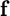with respect to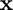at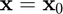using the complex-step approximation with a default step size of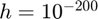.

J = ijacobian(f,x0,h) numerically evaluates the Jacobian ofwith respect toatusing the complex-step approximation with a user-specified step size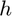.

## Input/Output Parameters

 Variable Symbol Description Format Input f multivariate, vector-valued function () 1×1function_handle x0 evaluation point n×1double h (OPTIONAL) step size 1×1double Output J Jacobian of with respect to , evaluated at m×ndouble

## Note

• This function requires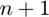evaluations of.

## Example

Approximate the Jacobian ofat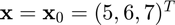using the ijacobian function, and compare the result to the true result of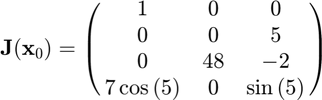Approximating the Jacobian,

f = @(x) [x(1);5*x(3);4*x(2)^2-2*x(3);x(3)*sin(x(1))];
x0 = [5;6;7];
J = ijacobian(f,x0)

J =

1.0000         0         0
0         0    5.0000
0   48.0000   -2.0000
1.9856         0   -0.9589



Calculating the error,

error = J-[1,0,0;0,0,5;0,48,-02;7*cos(5),0,sin(5)]

error =

0     0     0
0     0     0
0     0     0
0     0     0



NOTE: The function and its corresponding Jacobian are from an example on Wikipedia.